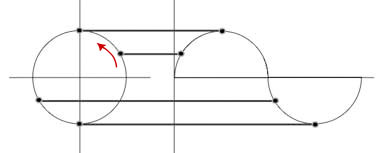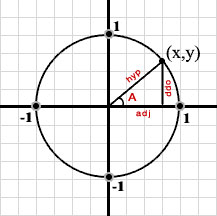Trigonometric Functions

# Wave Mathematics: Trigonometric Functions

by Gary Leo Welz, M.A./M.S.

Waves are familiar to us from the ocean, the study of sound, earthquakes, and other natural phenomenon. But as any surfer can tell you, ocean waves come in very different sizes, as can all waves. To fully understand waves, we need to understand measurements associated with these waves, such as how often they repeat (their frequency), and how long they are (their wavelength), and their vertical size (amplitude).

While these measurements help describe waves, they do not help us make predictions about wave behavior. In order to do that, we need to look at waves more abstractly, which we can do using a mathematical formula.

## Waves and circles

It is possible to look at waves mathematically because a wave's shape repeats itself over a consistent interval of time and distance. This behavior mirrors the repetition of the circle. Imagine drawing a circle on a piece of paper. Now imagine drawing that same shape while your friend slowly pulled the piece of paper out from under your pencil – the line you would have drawn traces out the shape of a wave. One rotation around the circle completes one cycle of rising and falling in the wave, as seen in the picture below.Figure 1: Circle on a cartesian plane.

Mathematicians use the sine function (Sin) to express the shape of a wave. The mathematical equation representing the simplest wave looks like this:

y = Sin(x)

This equation describes how a wave would be plotted on a graph, stating that y (the value of the vertical coordinate on the graph) is a function of the sine of the number x (the horizontal coordinate).

The sine function is one of the trigonometric ratios originally calculated by the astronomer Hipparchus of Nicaea in the second century B.C. when he was trying to make sense of the movement of the stars and moon in the night sky. More than 2000 years ago, when Hipparchus began to study astronomy, the movement of objects in the sky was a mystery. Hipparchus knew that the stars and moon tended to move through the night sky in a semi-circular fashion. Thus he felt that understanding the shape of a circle was important to understanding astronomy. Hipparchus began to observe that there was a relationship between the radius of a circle, the center angle made by a pie slice of that circle, and the length of the arc of that pie piece. If one knew any two of these values, the third value could be calculated. It was later realized that this relationship also applies to right triangles. Knowing one angle measure of a right triangle, you can calculate the ratio of the sides of the triangle. The exact size of the triangle can vary, but the ratio of the lengths of the sides is defined by the angle size. The specific relationships between the angle measure and the sides of the triangle are what we call the trigonometric functions, the three main functions consisting of:

• Sine A = opposite/hypotenuse
• Cosine A = adjacent/hypotenuse
• Tangent A = opposite/adjacent

The word trigonometry means "measurement of triangles" and sine along with the cosine and tangent are called the trigonometric ratios because they originated with the ancient study of triangles.

Comprehension Checkpoint

The ancient astronomer Hipparchus discovered that knowing one angle measure of a right triangle allows you to calculate

## Trigonometric ratios become wave functions

But how do triangles relate to waves? In the early 17th century, two Frenchmen named Rene Descartes and Pierre Fermat co-developed what would become known as the Cartesian coordinate plane, more commonly known as the (x,y)-graphing plane. This invention was an extraordinary advance in the history of mathematics because it brought together, for the first time, the integration of the two great, but distinct branches of mathematics: geometry, the science of space and form, and algebra, the science of numbers. The invention of the Cartesian coordinate system soon led to the graphing of many mathematical relations including the sine and cosine ratios.

As it turns out, the trigonometric functions can also be defined in relation to the "unit circle," i.e. a circle with a radius equal to 1. When we put the unit circle on the Cartesian plane, we can begin to see how this works if we draw a triangle within the circle, as seen in the diagram below. According to our earlier discussion, the sine of angle A in the diagram equals the ratio of the opposite side over the hypotenuse. However, remember that we are working with a unit circle and the length of the hypotenuse is equal to the radius of the circle, or 1. Therefore,

Sin(A) = opp/1 = opp

So the sine of A gives the length of the opposite side of the triangle, or the y-coordinate on our Cartesian plane. Similarly, the cosine of angle A equals the ratio of the adjacent side over the hypotenuse. Since the length of the hypotenuse equals 1, the cosine of A gives the length of the adjacent side, or the x-coordinate on the Cartesian plane.Figure 3: Shows the unit circle on the Cartesian Plane with an inscribed triangle. The point on the circle touched by the radius has coordinates (x,y).

If we redraw this triangle as we move counterclockwise on the circle, we can begin to see that the trigonometric functions, in this case sine and cosine, take on a periodic quality. This means that sine, for example, increases to a maximum at the top of the circle, decreases to zero as we sweep left, and begins to take on negative values as we continue around the circle. At the bottom of the circle the sine function reaches a minimum value and the process begins again as we reach the right side of the circle. To better appreciate this idea, review the animation Sine, Cosine, and the Unit Circle linked below.

Sine, Cosine, and the Unit Circle
This animation illustrates how the values of the sine and cosine change as we sweep around the unit circle.

As you saw in the animation above, as angle A increases, the values of the trigonometric functions of A undergo a periodic cycle from 0, to a maximum of 1, down to a minimum of -1, and back to 0. There are several ways to express the measure of the angle A. One way is in degrees, where 360 degrees defines a complete circle. Another way to measure angles is in a unit called the radian, where 2π radians defines a complete circle. Angles smaller than 360 can be defined as fractions of this unit, for example 90° can be written as π/2 or 1.57 radians, 180° equals π or 3.14 radians.

Comprehension Checkpoint

The measure of angles can be expressed in degrees or in

## Plotting a basic sine wave

If we now plot the sine of the angle measured in radians along the Cartesian coordinate system, we see that we again get the characteristic rise and fall. However, since the angle measure is plotted along the x-axis (instead of the cosine of the angle), the graph that results is a continuous curve on the coordinate plane that resembles a physical wave, as seen below.

If you look closely at this graph you will see that the wave crosses the x-axis at multiples of 3.1416 - the value of pi. One full wave is completed at the value 6.2832, or 2π, exactly the circumference of the unit circle.

Understanding the origin of the sine function makes it easier to understand how it operates in relation to waves. As we saw earlier, the basic formula representing the sine function is:

y = Sin(x)

In this formula, y is the value on the y-axis obtained when one carries out the function Sin(x) for points on the x-axis. This results in the graph of the basic sine wave. But how can we represent other forms of waves, especially ones that are larger or longer? To graph waves of different sizes we need to add other terms to our formula. The first we will look at is amplitude.

y = ASin(x)

In this modification of the formula, A gives us the value of the amplitude of the wave – the distance it moves above and below the x-axis, or the height of the wave. In essence, what the modifier A does is increase (or amplify) the result of the function Sin(x), thus leading to larger resulting y values.

To modify the wavelength of a wave, or the distance from one point on a wave to an equal point on the following wave, the modifier k is used, as seen in the formula below.

y = ASin(k*x)

The multiplier k extends the length of the wave. Remember from our earlier discussion that the wavelength of our most simple wave is 2π, therefore wavelength in the final formula is determined simply be dividing 2π by the multiplier k, so wavelength (λ) = 2π/k.

Comprehension Checkpoint

The multiplier k is used to modify the _____ of a wave.

## Wave periods

Since waves always are moving, one more important term to describe a wave is the time it takes for one wavelength to pass a specific point in space. This term, referred to as the period, T, is equivalent to the wavelength, T = Period = 2π/k, however it is given in units of time (sec) rather than distance.

Understanding the mathematics behind wave functions allows us to better understand the natural world around us. For example, the differences between the colors you see on this page have to do with different wavelengths of light perceived by your eyes. Similarly, the difference between a bird?s song and the roar of a locomotive is due to the size of the sound waves emitted. Waves, and thus the mathematics of waves, constantly surround us.

### Summary

Waves, circles, and triangles are closely related. In fact, this relatedness forms the basis of trigonometry. Basic trigonometric functions are explained in this module and applied to describe wave behavior. The module presents Cartesian coordinate (x, y) graphing, and shows how the sine function is used to plot a wave on a graph.

### Key Concepts

• The sine function is one of many trigonometric ratios calculated by astronomer Hipparchus over 2,000 years ago.

• Understanding trigonometric functions allows for the understanding and prediction of an object’s movement.

• ##### NGSS
• HS-C3.5, HS-PS4.A1

Gary Leo Welz, M.A./M.S. “Wave Mathematics” Visionlearning Vol. MAT-1 (1), 2006.

Top